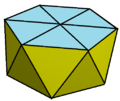# Hexagonal antiprism

Uniform Hexagonal antiprism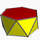TypePrismatic uniform polyhedron
ElementsF = 14, E = 24
V = 12 (χ = 2)
Faces by sides12{3}+2{6}
Schläfli symbols{2,12}
sr{2,6}
Wythoff symbol| 2 2 6
Coxeter diagram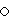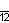Symmetry groupD6d, [2+,12], (2*6), order 24
Rotation groupD6, [6,2]+, (622), order 12
ReferencesU77(d)
DualHexagonal trapezohedron
Propertiesconvex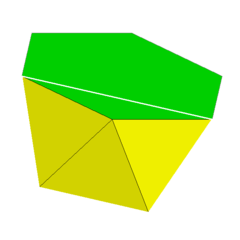Vertex figure
3.3.3.6

In geometry, the hexagonal antiprism is the 4th in an infinite set of antiprisms formed by an even-numbered sequence of triangle sides closed by two polygon caps.

Antiprisms are similar to prisms except the bases are twisted relative to each other, and that the side faces are triangles, rather than quadrilaterals.

In the case of a regular 6-sided base, one usually considers the case where its copy is twisted by an angle 180°/n. Extra regularity is obtained by the line connecting the base centers being perpendicular to the base planes, making it a right antiprism. As faces, it has the two n-gonal bases and, connecting those bases, 2n isosceles triangles.

If faces are all regular, it is a semiregular polyhedron.

The hexagonal faces can be replaced by coplanar triangles, leading to a nonconvex polyhedron with 24 equilateral triangles.